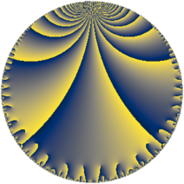# Properties

 Label 32.7.dLevel $32$ Weight $7$ Character orbit 32.d Rep. character $\chi_{32}(15,\cdot)$ Character field $\Q$ Dimension $5$ Newform subspaces $2$ Sturm bound $28$ Trace bound $1$

# Related objects

## Defining parameters

 Level: $$N$$ $$=$$ $$32 = 2^{5}$$ Weight: $$k$$ $$=$$ $$7$$ Character orbit: $$[\chi]$$ $$=$$ 32.d (of order $$2$$ and degree $$1$$) Character conductor: $$\operatorname{cond}(\chi)$$ $$=$$ $$8$$ Character field: $$\Q$$ Newform subspaces: $$2$$ Sturm bound: $$28$$ Trace bound: $$1$$ Distinguishing $$T_p$$: $$3$$

## Dimensions

The following table gives the dimensions of various subspaces of $$M_{7}(32, [\chi])$$.

Total New Old
Modular forms 28 7 21
Cusp forms 20 5 15
Eisenstein series 8 2 6

## Trace form

 $$5 q + 2 q^{3} + 727 q^{9} + O(q^{10})$$ $$5 q + 2 q^{3} + 727 q^{9} + 1362 q^{11} + 2442 q^{17} + 3938 q^{19} - 8275 q^{25} - 32860 q^{27} - 23500 q^{33} + 49920 q^{35} + 16698 q^{41} - 122542 q^{43} + 119765 q^{49} + 465412 q^{51} + 12500 q^{57} - 846990 q^{59} - 205440 q^{65} + 1386818 q^{67} - 149222 q^{73} - 2483950 q^{75} - 186839 q^{81} + 2786082 q^{83} + 403962 q^{89} - 3398400 q^{91} + 895978 q^{97} + 5702086 q^{99} + O(q^{100})$$

## Decomposition of $$S_{7}^{\mathrm{new}}(32, [\chi])$$ into newform subspaces

Label Dim $A$ Field CM Traces $q$-expansion
$a_{2}$ $a_{3}$ $a_{5}$ $a_{7}$
32.7.d.a $1$ $7.362$ $$\Q$$ $$\Q(\sqrt{-2})$$ $$0$$ $$-46$$ $$0$$ $$0$$ $$q-46q^{3}+1387q^{9}+2338q^{11}-1726q^{17}+\cdots$$
32.7.d.b $4$ $7.362$ 4.0.3803625.2 None $$0$$ $$48$$ $$0$$ $$0$$ $$q+(12-\beta _{1})q^{3}-\beta _{2}q^{5}+(\beta _{2}-\beta _{3})q^{7}+\cdots$$

## Decomposition of $$S_{7}^{\mathrm{old}}(32, [\chi])$$ into lower level spaces

$$S_{7}^{\mathrm{old}}(32, [\chi]) \cong$$ $$S_{7}^{\mathrm{new}}(8, [\chi])$$$$^{\oplus 3}$$